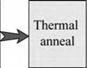## Doping activation in III-V nitrides

Another problem in III-V nitrides is the low doping activation, which is caused by two effects: (i) Chemical deactivation of acceptors by hydrogen atoms bonding to the acceptors. The missing electron that acceptors strive to capture is provided by a hydrogen atom. Hydrogen is available in abundance during epitaxial growth and possible sources for hydrogen passivation include the methyl (-CH3) and ethyl groups (-C2H5) of the organo-metallics, ammonia (NH3), and hydrogen from the H2 carrier gas. (ii) Acceptors in III-V nitrides have a high thermal activation energy which is >> kT at 300 K. As a result, only a small percentage of acceptors are ionized at room temperature.

Exercise: Activation of Mg acceptors in GaN. Mg acceptors in GaN have an activation energy of Ea = 200 meV. (a) Calculate the fraction of acceptors that are ionized at 300 K for an acceptor concentration of NMg = 1018 cm3 using the formula p = (g4 NMg Nv)1/2 exp (- Ea / 2kT) where g is the acceptor ground state degeneracy (g = 4) and Nv is the effective density of states at the valence band edge of GaN. (b) What would be the activation of acceptors if a hydrogen atom were bonded to each acceptor?

Solution: (a) Using the formula given above, one obtains that only about 6% of the acceptors are ionized. (b) If acceptors are passivated, p-type conductivity cannot be established.

Amano et al. (1989) discovered that acceptor dopants can be activated by low-energy electron-beam irradiation (LEEBI). Nakamura et al. (1991; 1992) showed that acceptors can be activated by LEEBI as well as by thermal annealing, with thermal annealing being the preferred method. Typical annealing conditions for MOCVD-grown p-type GaN are 675-725 °C for 5 minutes in an N2 atmosphere; p-type Al0.30Ga0.70N is annealed at higher temperatures, typically at 850 °C for 1-2 minutes. It is believed that during thermal annealing the relatively weak acceptor-hydrogen bond is broken and that the hydrogen atoms are driven out of the epitaxial film. Hydrogen atoms are small and generally diffuse easily through the interstitial sites of a crystalline material. The heating caused by LEEBI is believed to have a similar effect.Acceptors passivated by H2

The doping of AlGaN/GaN and AlGaN/AlGaN superlattices (rather than GaN or AlGaN bulk material) was postulated to strongly increase the electrical activation of deep acceptors such as Mg (Schubert et al., 1996). Experimental increases in conductivity by a factor of 10 and more have indeed been reported by several research groups (Goepfert et al., 1999, 2000; Kozodoy et al. 1999a, b; Kipshidze et al., 2002, 2003). Figure 13.6 summarizes the problems and solutions to the doping activation in GaN and AlGaN.

 Super­ lattice doping
 Low activation due to high acceptor ionization energy

 Fig. 13.6. Specific problems of p-type doping in GaN and their solution.

Комментарии закрыты.